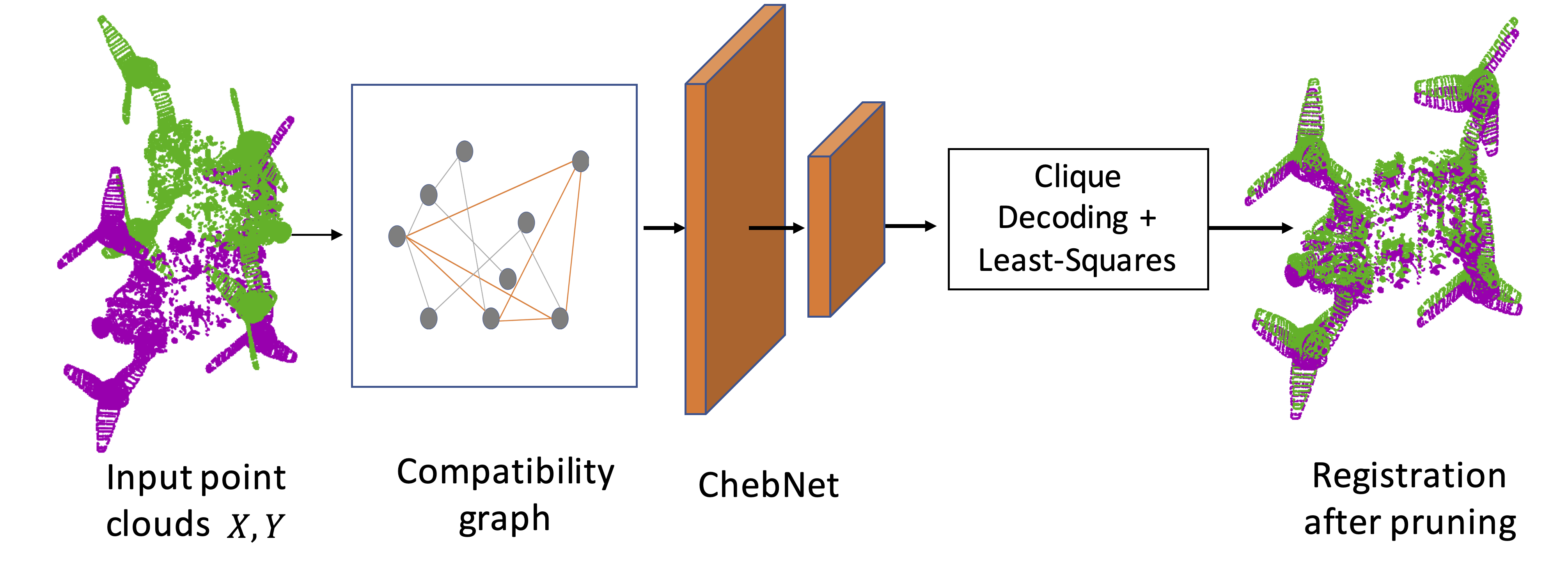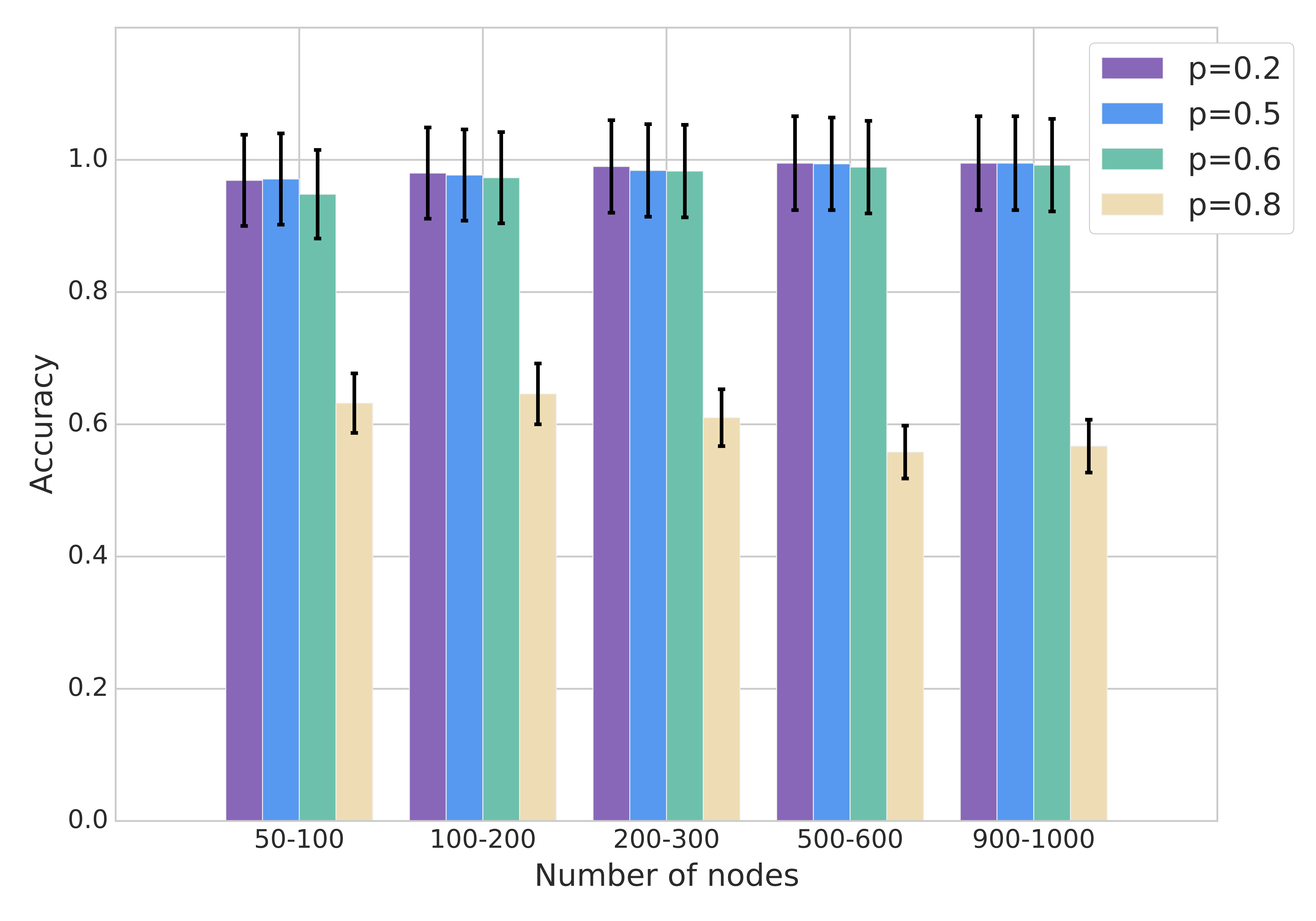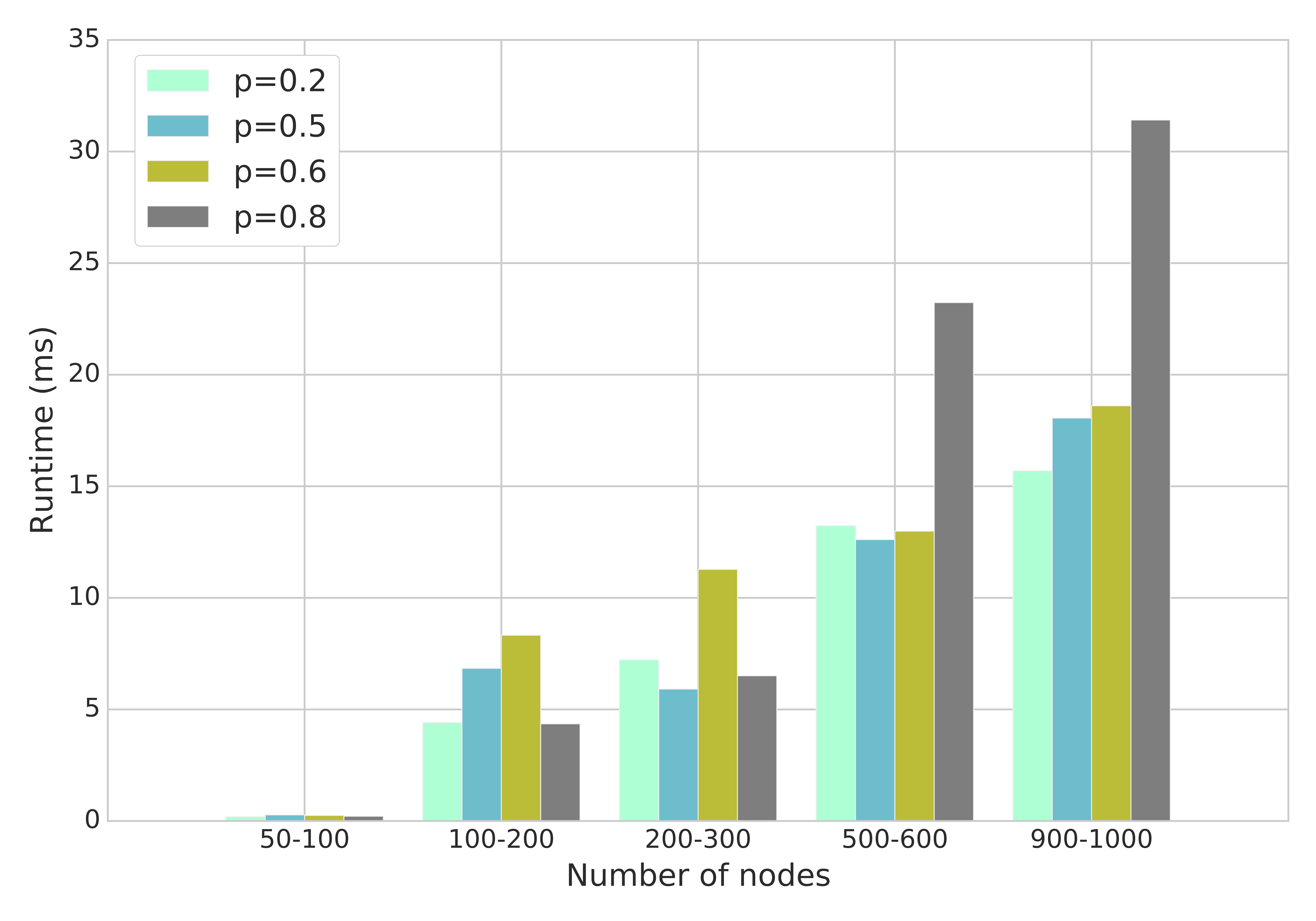### Robust 6D Object Pose Estimation

Pose estimation is critical in many robotics applications, particularly to enable autonomous vehicles to perceive other vehicles around them. We design algorithms that allow 6D pose estimation in challenging scenarios, including heavy occlusion, while under computational constraints.

## Robust Object Pose Estimation

### Background

Robust estimation is the problem of detecting and removing spurious data points from outlier-contaminated data. The problem is unavoidable in computer vision and robotics applications due to imperfect visual data and error-prone preprocessing (e.g feature extraction). It is thus a key component of algorithms for numerous vision tasks, such as lane tracking in autonomous driving, 6-DoF object pose es- timation in robotic manipulation, and structure from motion in multi-view geometry.

Exact algorithms such as mixed integer programming and branch-and-bound are thus computationally infeasible in real applications. While fast approximate algorithms exist, there is a lack of analysis on when such algorithms break down.

### The Planted-Clique Perspective

Robust estimation is often formulated as consensus maximization on input data. We can decouple the problem into two subproblems taking advantage of pairwise consistency of geometric data. We first search for pairwise consistent hypotheses resulting in an outlier-free inlier set. It is then trivial to find the exact solution to the estimation problem on the found inlier set. The searching problem is often framed as the maximum clique problem in the literature and an exact search-based solver can be applied to find the optimal solution. However, exact solvers are too computationally expensive to be applied on real data.

Motivated by the aforementioned observations and recent advances of planted clique algorithms, we propose an alternative paradigm for hypothesis pruning in a learning framework. We translate geometric perception problems, e.g., point cloud registration, to a search problem of planted clique and then train a graph neural network (GNN) model to learn clique structures in simple random graphs. Reliable estimation from learning results is a challenging problem. We address this challenge by iteratively reconstructing the inlier set from heuristic predictions of GNNs.

We address this challenge by iteratively reconstructing the inlier set from heuristic predictions of GNNs. The following figure describes our approach. A pair of input point clouds with corrupted initial correspondences is used to create a compatibility graph. We then run an off-the-shelf ChebNet on the compatibility graph to obtain a probability estimation of being an inlier for each pair of correspondence. Our approach then iteratively decodes a valid clique for the final registration.### Results

By drawing the connection between hypothesis pruning and the planted clique problem, we show that while no efficient solvers exist in worst cases, fast heuristics recover the optimal solution under certain conditions. We then design an efficient algorithm based on learned heuristic and show its effectiveness on vision problems. We present experiments on both synthetic and real datasets to verify our findings. The proposed algorithm achieves competitive results and runs much faster than the exact solver and existing heuristics.

The proposed algorithm achieves competitive results and runs much faster than the exact solver and existing heuristics. The figure below shows that the trained graph neural network model generalizes to random graphs of different distributions. In the figure, p denotes the probability of each edge being included in a random graph (larger p yields larger cliques formed by outliers).We present the following contributions: 1) We show the information-theoretical and computational limits of the hypothesis pruning problem via its connection to the planted clique model. The connection indicates that no efficient algorithms exist when the cardinality of the inlier set is significantly below √n. 2) We also propose a learning framework that trains a graph neural network on synthetic random graphs with planted cliques. By focusing on pairwise relationship of clique pat- tern, the training avoids overfitting against task-specific node features, and consequently the trained model generalizes to unseen real datasets with a large runtime improvement.

### Software

Software implementation is available open source via the MIT License.